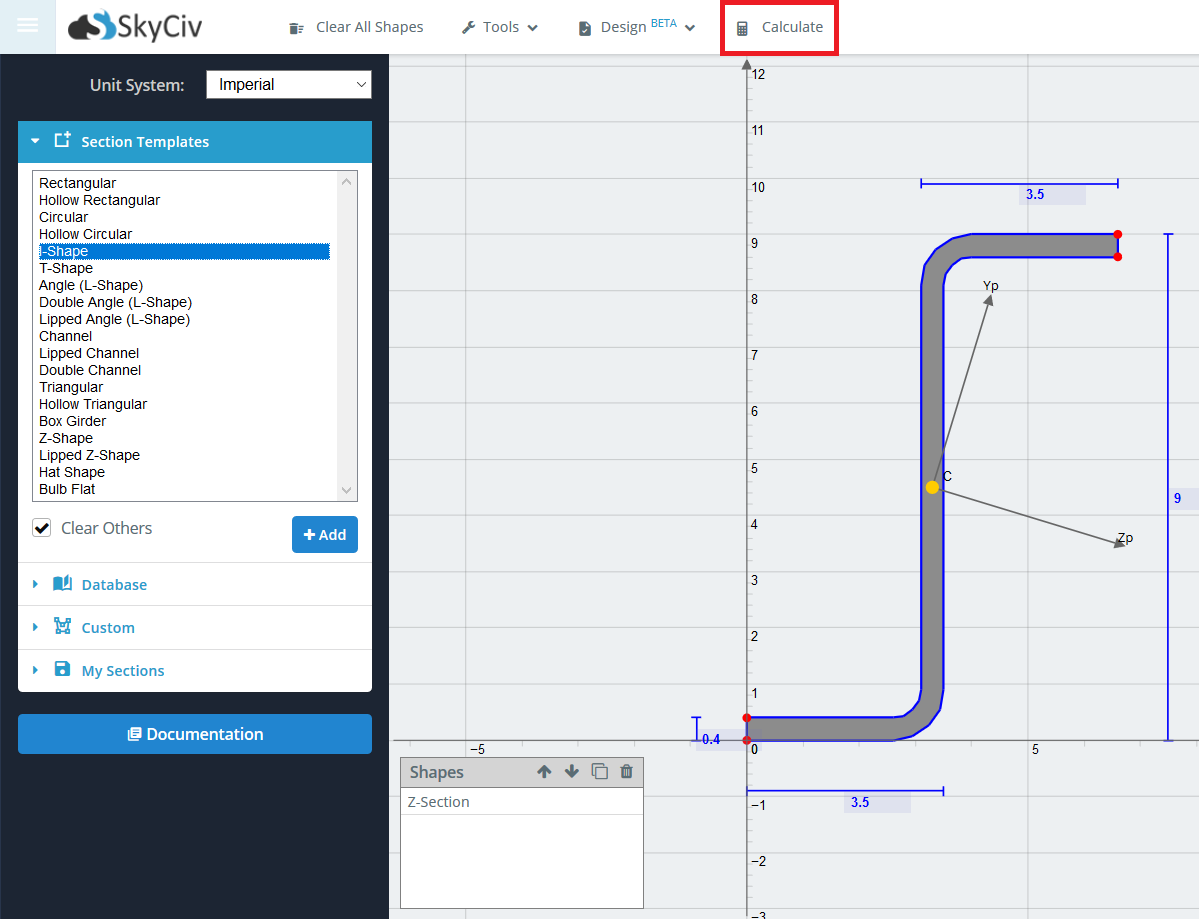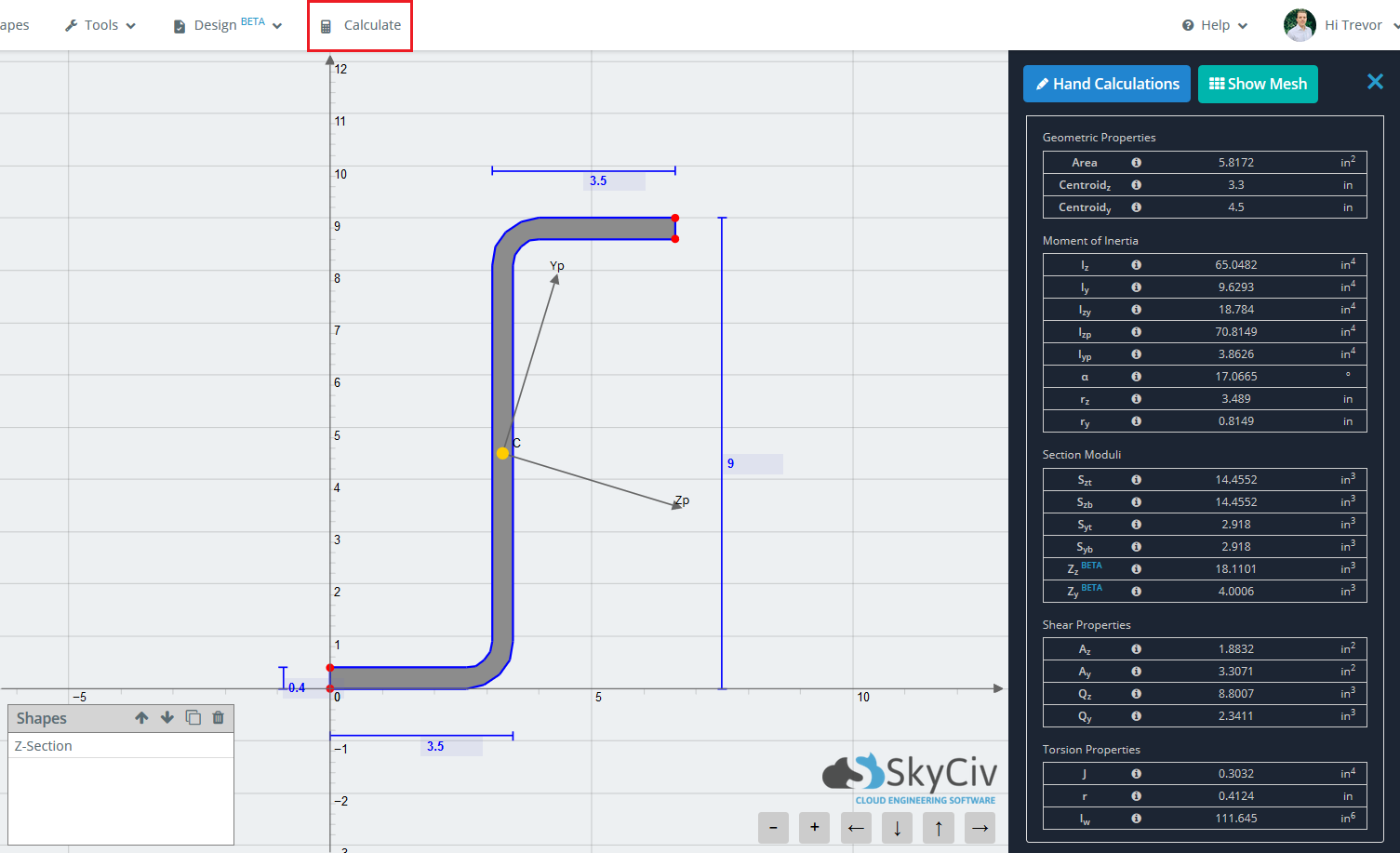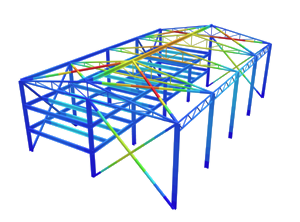SkyCiv Documentation

Your guide to SkyCiv software - tutorials, how-to guides and technical articles

1. Home
2. SkyCiv Section Builder
3. Results
4. Standard Results

# Standard Results

The main purpose of the Section Builder is to calculate the various section properties of your section. The standard results refer to the results of a section when ignoring material property differences.

When you’ve finished building your section, simply click ‘Calculate’ on the top toolbar to determine the section properties. Note that the short wait time associated with each calculate is due to the Finite Element Analysis and Meshing that occurs in the background in order to solve for torsion constant, torsion radius, and warping constant. By using a FEA method to calculate these properties, a higher accuracy is achieved. Less robust programs only use approximate equations on the standard sections for these properties and fail to solve when a non standard section (such as the cross beam below) is entered.The results are generally broken down into 5 main categories.

• Geometric Properties
• Area
• Centroid (Z)
• Centroid (Y)
• Moment of Inertia Properties
• Iz – Moment of Inertia about the Z-axis
• Iy – Moment of Inertia about the Y-axis
• Izy – Moment of Inertia
• Izp – Moment of Inertia about the principal Z-axis
• Iyp – Moment of Inertia about the principal Y-axis
• α – angle of rotation of principal axis
• Section Moduli
• Szt – Elastic Section Modulus about the Z-axis to the top of the section
• Szb – Elastic Section Modulus about the Z-axis to the bottom of the section
• Syt – Elastic Section Modulus about the Y-axis to the top of the section
• Syb – Elastic Section Modulus about the Y-axis to the bottom of the section
• Zz – Plastic Section Modulus about the Z-axis
• Zy – Plastic Section Modulus about the Y-axis
• Shear Properties
• Az – Shear Area about Z axis
• Ay – Shear Area about Y axis
• Qz – Static Moment of Area about the Z neutral axis
• Qy – Static Moment of Area about the Y neutral axis
• Torsion Properties
• J – Torsion Constant